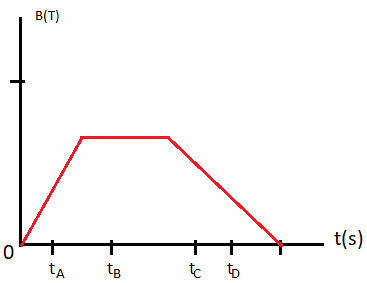# The figure below shows the time evolution of a uniform magnetic field. Four particular instants...

## Question:

The figure below shows the time evolution of a uniform magnetic field. Four particular instants labeled t{eq}_A {/eq} to t{eq}_D {/eq} are also identified on the graph. The field passes through a circular coil whose normal is parallel to the direction of the field. At what time does the current induced in the coil have largest value?A) t{eq}_A {/eq}

B) t{eq}_B {/eq}

C) t{eq}_C {/eq}

D) t{eq}_D {/eq}

E) The current is the same at all these times

Faraday's law dictates the strength of the electric field induced in a region when the magnetic field strength (more generally, the magnetic flux in the region is varying). According to Faraday's law, when magnetic flux through a loop varies, it induces an emf in the loop whose magnitude is given by:

{eq}E \ = \ \dfrac{d \phi}{dt} {/eq}

Where:

{eq}\phi \ = \ \vec{B} \ \cdot \ \vec{A} {/eq} - is the magnetic flux through the loop

Let {eq}B(t) {/eq} be the magnetic field at any given instant {eq}t {/eq}. Amount of magnetic flux passing through the circular loop is given by: {eq}\phi \ = \ B(t) \times A {/eq}

Where:

{eq}A {/eq} - is the area of the circular element

Rate of change of magnetic flux through the loop is given by: {eq}\dfrac{d \phi}{dt} \ = \ \dfrac{d B(t)}{dt} \times A {/eq}

From Faraday's law, magnitude of emf induced in the loop equals the rate of change of magnetic flux through the loop i.e.

{eq}E \ = \ \dfrac{d\phi}{dt} \ = \ \dfrac{d B(t)}{dt} \times A {/eq}

Induced emf (and hence the induced current) is maximum when {eq}\dfrac{d B(t)}{dt} {/eq} is maximum. This happens for {eq}t \ = \ t_A {/eq} in the figure given.

Therefore, the correct answer is option A.